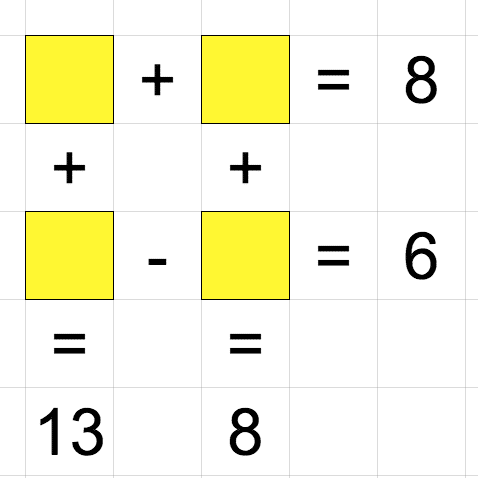# Easy Puzzles

Lecture 3 : 27 April 2020

Instead of a lecture, we will solve some simple puzzles using linear algebra. We will only need one technique from the first lecture: the inverse of a matrix.

## Review: Matrix inverse

Recall that if we wish to solve for the vector \$x\$ in the matrix equation \$Ax = b\$, we can find the inverse \$A^{-1}\$ then multiply it with the given vector \$b\$.

We can do this in Python with `np.linalg.inv(A)`. Let’s see a full example, using \$A\$ and \$b\$ from lecture 1.

We’ve discussed almost everything in this code except `b = np.array([16,-5,-3])` in line 7. This is how we define vectors in Python. Notice that we only use one set of square brackets.

If you want, you can also check that your solution works, by computing `A @ x` and comparing it with `b`.

## Instructions

For each of the puzzles below, copy the above code into your Spyder editor and modify `A` and `b` to suit your problem. The point is to get used to setting up the puzzle as a matrix equation, and defining the matrix in Python, not so much in finding the answer.

You can use a separate file for each puzzle, or put them in the same file. If you put them in the same file, try to use different names for `A` and `b` in each puzzle. Email me your code for at least 2 of the puzzles below.

## 1. Square Dance

Solve for the unknowns in the yellow squares:If you’ve defined `A` for the above puzzle, you only need to change `b` to solve this:## 2. Triangles

The numbers in each square are the sums of the numbers in their adjacent circles. Can you figure out the numbers in the circles?Again, it’s more important to set up the matrix `A` than to actually find solutions to all three triangles.

Bonus: Does our method work for squares? Pentagons? Hexagons? Can you guess which polygons it works for?## 3. Animal Crossing

Only do this if you’re very free. The solution is already there, so the important part is not finding the solution, but setting up the matrix. The numbers on the sides are the sums of the respective rows and columns.

Hint: If there are too many equations to form a square matrix, try dropping one of them.## 4. Viral Fruit

Variants of this puzzle are being passed around online. But the tricky part is not the linear algebra!## Next time…

You might have found it quite tedious to define some of these matrices. For most of these puzzles, we don’t really have a choice, as the matrices involved are specific to that puzzle.

But for Puzzle 3, we can use Python functions and loops to quickly define matrices for any polygon. We will learn about functions and loops next time, and use them to solve puzzles like the following:

What’s the next number in the series: 1,6,23,58,__ ?